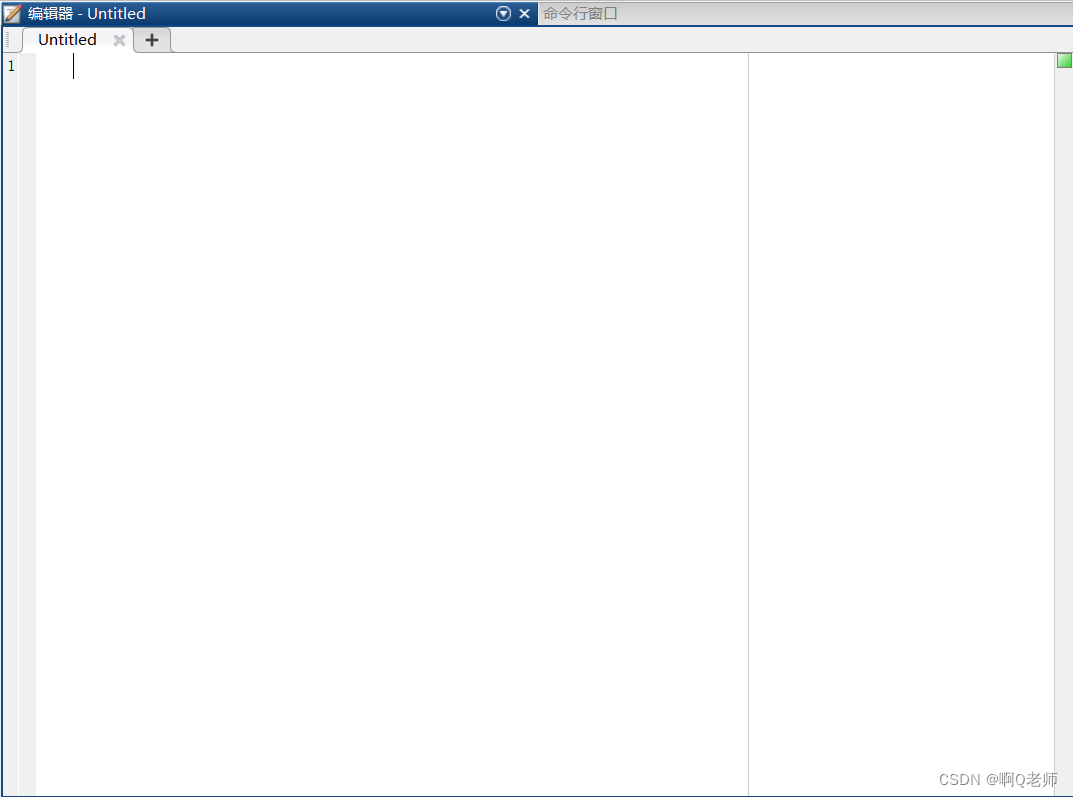# 脚本

Matlab命令执行方式有交互式的命令执行与脚本两种方式。交互式的命令执行方式，在命令行窗口逐条输入命令，MATLAB逐条解释执行；而脚本方式，将有关命令编成程序存储在一个扩展名为.m的文件中，每次运行该脚本，Matlab就会自动依次执行脚本中的命令。

ui

## 脚本的编辑Wi-Fi

• 点击Matlab桌面的“主页”，点击选项卡工具条中的“新建脚本”
• Matlab命令行窗口中输入命令：
edit 文件名

``````%示例：在指定的Matlab工作目录下，对命令窗口进行输入：
>> edit setcomp
%创建文件名后会自动跳转在编辑器下，然后输入如下代码并保存：

R=[1,2,3;4,5,6];
M=[11,12,13;14,15,16];
RM=complex(R,M);

>> %再在命令行窗口中输入脚本文件名，按Enter运行：
>> setcomp
>>%运行后，再输入RM，查看结果：
>> RM

RM =

1.0000 +11.0000i   2.0000 +12.0000i   3.0000 +13.0000i
4.0000 +14.0000i   5.0000 +15.0000i   6.0000 +16.0000i

``````

# 程序控制结构

cuda

## 顺序结构

input()函数：通过键盘的输入获取数据

disp()函数：用于在命令窗口显示变量和表达式的值

``````%示例：
>> matrix1 = input('创建2x3阶元素全为1的矩阵：')

matrix1 =

1     1     1
1     1     1

>> name = input('请输入你的名字：')

name =

'小明'

>> %或者：
>>  name = input('请输入你的名字：','s')

name =

'小明'

>> disp(matrix1)
1     1     1
1     1     1

>> disp(name)

``````

## 选择结构

jsf

``````(1)单分支：
if  条件表达式
语句块

(2)双分支：
if  条件表达式1
语句块1
else
语句块2

(3)多分支：
if  条件表达式1
语句块1
elseif  条件表达式2
语句块2
…
elseif  条件表达式n
语句块n
else
语句块n+1
end
``````
``````%在指定的Matlab工作目录下，对命令窗口进行输入：
>> edit opt %创建脚本
%创建文件名后会自动跳转在编辑器下，然后输入如下代码并保存：

%示例：
%输入一个字符，若为大写字母，则输出其对应的小写字母；
%若为小写字母，则输出其对应的大写字母；
%若为数字字符则输出其对应数的平方，若为其他字符则原样输出。
str = input("请输入一个字符：","s");
if str >= 'A' && str <= 'Z'
disp(lower(str));
elseif str >= 'a' && str <= 'z'
disp(upper(str));
elseif str >= '0' && str <= '9'
disp(str2double(str)^2);
else
disp(str);
end

>> %再在命令行窗口中输入脚本文件名，按Enter运行：
>> edit opt
>> opt

s
``````

## 循环结构

ccna

``````break语句用于终止循环的执行；continue语句控制跳过循环体中的某些语句。
(1)for语句：
for 循环变量=表达式1:表达式2:表达式3
循环体语句
end

(2)while语句：
while 条件表达式
循环体语句
end
``````
``````%在指定的Matlab工作目录下，对命令窗口进行输入：
>> edit NarcissisticNumber %创建脚本
%创建文件名后会自动跳转在编辑器下，然后输入如下代码并保存：

%示例：
%一个各位数字的立方和等于该数本身的3位整数称为水仙花数。输出全部水仙花数
Nar_num = []; %创建空矩阵存放结果
for n = 100:999
bai = fix(n/100);            	%求n的百位数字
shi = mod(fix(n/10),10);    	%求n的十位数字
ge = mod(n,10);             	%求n的个位数字
if n == bai^3+ shi^3 + ge^3
Nar_num=[Nar_num,n];             	%存入结果
end
end
disp(Nar_num)

>> %再在命令行窗口中输入脚本文件名，按Enter运行：
>> NarcissisticNumber
153   370   371   407
``````

# 函数

sql优化

## 函数文件

1.函数名的命名规则与变量名的命名规则相同；
2.有多个参数时，参数和参数之间用逗号分隔；
3.若输出参数多于一个，则应该用方括号括起来。

function 输出形参表=函数名(输入形参表)

inotify

[输出实参表]=函数名(输入实参表)

ConcurrenMap

``````%点击"新建"，再点击"函数"，创建函数文件
%编写函数文件，求1/(m+1)+1/(m+2)+…+1/(m+n)与1/m(m+1)+1/(m+1)(m+2)+…+1/(m+n−1)(m+n)。
function [s1,s2]=sumfraction(m,n)
%SUM  sumfraction.m calculates sum of fractions
%m          区间下界
%n          区间上界
%s1         1/(m+1)+1/(m+2)+...+1/(m+n)
%s2         1/m/(m+1)+1/(m+1)/(m+2)+...+1/(m+n-1)/(m+n)
s1=0;s2=0;
for k=m+1:m+n
s1=s1+1/k;
s2=s2+1/(k-1)/k;
end

>> %在命令窗口调用以上定义的函数，示例：
>> %求1/(8+1)+1/(8+2)+…+1/(8+8)与1/8*(8+1)+1/(8+1)(8+2)+…+1/(8+8−1)(8+8)。
>> %形参m、n对应的值分别为8、8。
>> [s1,s2]=sumfraction(8,8)

s1 =

0.6629

s2 =

0.0625
``````

## 匿名函数

``````%示例：
>> func = @(x)8*x+8;
>> num = 88;
>> number = func(num)

number =

712
``````

# 经典案例——猴子吃桃子

``````%解答：
>> %普通循环算法：
>> x(1)=1;
>> for i=1:9
x(i+1)=2*x(i)+2;
end
>> x(10)

ans =

1534

>> %递归循环算法：
>> edit MonkeyEatSpeech

function [S] = MonkeyEatSpeech(x)
if x == 10
S = 1;
else
S = 2+2*MonkeyEatSpeech(x+1);
end

>> S = MonkeyEatSpeech(1)

S =

1534
``````